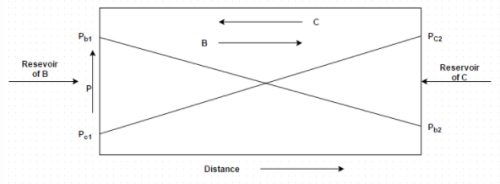Courses

# Test: Fick’s Law

## 20 Questions MCQ Test Mass Transfer | Test: Fick’s Law

Description
This mock test of Test: Fick’s Law for Chemical Engineering helps you for every Chemical Engineering entrance exam. This contains 20 Multiple Choice Questions for Chemical Engineering Test: Fick’s Law (mcq) to study with solutions a complete question bank. The solved questions answers in this Test: Fick’s Law quiz give you a good mix of easy questions and tough questions. Chemical Engineering students definitely take this Test: Fick’s Law exercise for a better result in the exam. You can find other Test: Fick’s Law extra questions, long questions & short questions for Chemical Engineering on EduRev as well by searching above.
QUESTION: 1

### Fick’s law is given by the formula

Solution:

Explanation: Experimental evidences shows that molecular diffusion is goverened by the empirical relation suggested by Fick.

QUESTION: 2

### Figure shows equimolal diffusion between species B and C. Identify the correct molar diffusion rateSolution:

Explanation: Equimolal diffusion between species B and C of a binary gas system implies that each molecule of component B is replaced by each molecule of constituent C.

QUESTION: 3

### What is the unit of diffusion coefficient?

Solution:

Explanation: D = – N/ (d C/ d x).

QUESTION: 4

What is the molecular weight of Sulphur-dioxide?

Solution:

Formula of Sulphur-dioxide is SO 2.

QUESTION: 5

What is the molecular volume of air at normal boiling point?

Solution:

Explanation: Air contains some dust particles due to which its molecular volume decreases by a small factor.

QUESTION: 6

What is the value of Schmidt number for hydrogen?

Solution:

Explanation: Schmidt number = v/D.

QUESTION: 7

Estimate the diffusion coefficient for ammonia in air t 25 degree Celsius temperature and one atmospheric pressure.
For ammonia,
Molecular weight = 17
Molecular volume = 25.81 cm3/gm mole
For air,
Molecular weight = 29

Solution:

Explanation: D = 0.0043 T 3/2/p t (V b1/3 + V c1/3[1/M + M c] ½.

QUESTION: 8

Estimate the diffusion coefficient of carbon monoxide through air in which the mole fractions of each constituents are
2 = 0.18
2 = 0.72
CO = 0.10
The gas mixture is at 300 K and 2 atmosphere total pressure. The diffusivity values are
CO = 18.5 * 10 -6 m2/s at 273 K, 1 atm
CN = 19.2 * 10 -6 m2/s at 288 K, 1 atm
The subscripts C is carbon monoxide, O is oxygen and N is nitrogen

Solution:

Explanation: (T 1/T 2) 3/2 (p 2/p 1) = D 1/D 2.

QUESTION: 9

What is the molecular weight of Sulphur-dioxide?

Solution:

Explanation: Formula of Sulphur-dioxide is SO 2.

QUESTION: 10

The dimension of diffusion coefficient is given by

Solution:

Explanation: Unit is m2/s

QUESTION: 11

For what kind of mixtures DAB=DBA holds?

Solution:

Explanation: None.

QUESTION: 12

In the expression for molar flux NA=(NA+NB)CA/C – DABdCA/dz, the terms representing bulk flow and molecular diffusion are, respectively

Solution:

Explanation: None.

QUESTION: 13

Consider loss of ethanol vapor by diffusion from a half-filled open test tube. At what point in the diffusion path will the contribution of the bulk flow term to the molar flux be maximum?

Solution:

Explanation: At the liquid-gas interface concentration will be maximum.
Bulk liquid is not a part of the diffusion path.

QUESTION: 14

A reaction 3A+2B+C=1.5D+E occurs in a reactor at steady state. What will be the value of the flux ratio NA/N

Solution:

Explanation: NA/ND= (rate of disappearance of A)/(rate of appearance of D)
= -3/1.5
= -2.

QUESTION: 15

For a component A of a mixture, concentration as a function of x is given:
CA=5e-10x (x is in cm and CA in mol/liter)
Calculate the value of diffusion velocity (m/s)of the component A at the point x=0, if diffusivity of A in the mixture is 2.567*10-5m2/s.

Solution:

Explanation: By Fick’s law, JA=(-)DAB*dC/dx
CA(uA-U)= (-)DABdC/dx…………1
Diffusion velocity= (uA-U)=(-DABdC/dx)/CA
dC/dx = -50e-10x
CA= 5 mol/lit
Put all the values in equation 1.

QUESTION: 16

A sheet of Fe 1.0 mm thick is exposed to a oxidizing gas on one side and a deoxidizing gas on the other at 725°C. After reaching steady state, the Fe membrane is exposed to room temperature, and the C concentrations at each side of the membrane are 0.012 and 0.075 wt%. Calculate the diffusion coefficient (m2/sec) if the diffusion flux is 1.4×10-8kg/m2-sec.

Solution:

Explanation: Convert from wt% to kg/m3 .
Concentration in kg/m3=[C1/(C11+C2/ρ2)]*1000
0.012% and 0.075% in kg/m3 are 0.270 and 1.688 respectively.
Putting all the values in the equation JA=(-)DABdC/dx.

QUESTION: 17

At which condition molar flux with respect to a stationary observer and with respect to a observer moving with molar average velocity?

Solution:

Explanation: In a very dilute solution, the contribution of the bulk flow term becomes negligible.
NA= (NA+NB)CA/C – DABdCA/dz (general equation)
(NA+NB)CA/C =0 (in dilute solution)
NA= -DABdCA/dz = JA.

QUESTION: 18

Which among the following is the statement of the ‘Fick’s Law’?

Solution:

Explanation: None.

QUESTION: 19

Fick’s law is given by the formula

Solution:

Experimental evidences shows that molecular diffusion is goverened by the empirical relation suggested by Fick.

QUESTION: 20

In industries titanium is hardened through diffusion of carbon. The concentration of carbon at 1mm into the surface of the titanium slab is 0.25kg/m3 and at 3mm the concentration is 0.68kg/m3. The rate at which the carbon is entering into its surface is 1.27*10-9kg/m2.s. calculate the value of diffusion coefficient of carbon.

Solution:

Explanation: J=-D(∂C/∂x)
∂C/∂x= Δc/Δx=(0.25-0.68)/(0.003-0.001)=-215kg/m4
1.27*10-19kg/m2.s= -D*(-215kg/m4)
D=5.91*10-12m2/s.